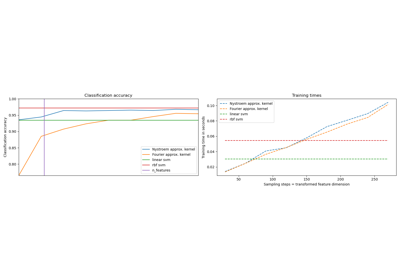# sklearn.kernel_approximation.RBFSampler¶

class sklearn.kernel_approximation.RBFSampler(*, gamma=1.0, n_components=100, random_state=None)[source]

Approximate a RBF kernel feature map using random Fourier features.

It implements a variant of Random Kitchen Sinks.

Read more in the User Guide.

Parameters:
gamma‘scale’ or float, default=1.0

Parameter of RBF kernel: exp(-gamma * x^2). If gamma='scale' is passed then it uses 1 / (n_features * X.var()) as value of gamma.

New in version 1.2: The option "scale" was added in 1.2.

n_componentsint, default=100

Number of Monte Carlo samples per original feature. Equals the dimensionality of the computed feature space.

random_stateint, RandomState instance or None, default=None

Pseudo-random number generator to control the generation of the random weights and random offset when fitting the training data. Pass an int for reproducible output across multiple function calls. See Glossary.

Attributes:
random_offset_ndarray of shape (n_components,), dtype={np.float64, np.float32}

Random offset used to compute the projection in the n_components dimensions of the feature space.

random_weights_ndarray of shape (n_features, n_components), dtype={np.float64, np.float32}

Random projection directions drawn from the Fourier transform of the RBF kernel.

n_features_in_int

Number of features seen during fit.

New in version 0.24.

feature_names_in_ndarray of shape (n_features_in_,)

Names of features seen during fit. Defined only when X has feature names that are all strings.

New in version 1.0.

AdditiveChi2Sampler

Approximate feature map for additive chi2 kernel.

Nystroem

Approximate a kernel map using a subset of the training data.

PolynomialCountSketch

Polynomial kernel approximation via Tensor Sketch.

SkewedChi2Sampler

Approximate feature map for “skewed chi-squared” kernel.

sklearn.metrics.pairwise.kernel_metrics

List of built-in kernels.

Notes

See “Random Features for Large-Scale Kernel Machines” by A. Rahimi and Benjamin Recht.

 “Weighted Sums of Random Kitchen Sinks: Replacing minimization with randomization in learning” by A. Rahimi and Benjamin Recht. (https://people.eecs.berkeley.edu/~brecht/papers/08.rah.rec.nips.pdf)

Examples

>>> from sklearn.kernel_approximation import RBFSampler
>>> from sklearn.linear_model import SGDClassifier
>>> X = [[0, 0], [1, 1], [1, 0], [0, 1]]
>>> y = [0, 0, 1, 1]
>>> rbf_feature = RBFSampler(gamma=1, random_state=1)
>>> X_features = rbf_feature.fit_transform(X)
>>> clf = SGDClassifier(max_iter=5, tol=1e-3)
>>> clf.fit(X_features, y)
SGDClassifier(max_iter=5)
>>> clf.score(X_features, y)
1.0


Methods

 fit(X[, y]) Fit the model with X. fit_transform(X[, y]) Fit to data, then transform it. get_feature_names_out([input_features]) Get output feature names for transformation. Get metadata routing of this object. get_params([deep]) Get parameters for this estimator. set_output(*[, transform]) Set output container. set_params(**params) Set the parameters of this estimator. Apply the approximate feature map to X.
fit(X, y=None)[source]

Fit the model with X.

Samples random projection according to n_features.

Parameters:
X{array-like, sparse matrix}, shape (n_samples, n_features)

Training data, where n_samples is the number of samples and n_features is the number of features.

yarray-like, shape (n_samples,) or (n_samples, n_outputs), default=None

Target values (None for unsupervised transformations).

Returns:
selfobject

Returns the instance itself.

fit_transform(X, y=None, **fit_params)[source]

Fit to data, then transform it.

Fits transformer to X and y with optional parameters fit_params and returns a transformed version of X.

Parameters:
Xarray-like of shape (n_samples, n_features)

Input samples.

yarray-like of shape (n_samples,) or (n_samples, n_outputs), default=None

Target values (None for unsupervised transformations).

**fit_paramsdict

Returns:
X_newndarray array of shape (n_samples, n_features_new)

Transformed array.

get_feature_names_out(input_features=None)[source]

Get output feature names for transformation.

The feature names out will prefixed by the lowercased class name. For example, if the transformer outputs 3 features, then the feature names out are: ["class_name0", "class_name1", "class_name2"].

Parameters:
input_featuresarray-like of str or None, default=None

Only used to validate feature names with the names seen in fit.

Returns:
feature_names_outndarray of str objects

Transformed feature names.

Get metadata routing of this object.

Please check User Guide on how the routing mechanism works.

Returns:

A MetadataRequest encapsulating routing information.

get_params(deep=True)[source]

Get parameters for this estimator.

Parameters:
deepbool, default=True

If True, will return the parameters for this estimator and contained subobjects that are estimators.

Returns:
paramsdict

Parameter names mapped to their values.

set_output(*, transform=None)[source]

Set output container.

See Introducing the set_output API for an example on how to use the API.

Parameters:
transform{“default”, “pandas”}, default=None

Configure output of transform and fit_transform.

• "default": Default output format of a transformer

• "pandas": DataFrame output

• None: Transform configuration is unchanged

Returns:
selfestimator instance

Estimator instance.

set_params(**params)[source]

Set the parameters of this estimator.

The method works on simple estimators as well as on nested objects (such as Pipeline). The latter have parameters of the form <component>__<parameter> so that it’s possible to update each component of a nested object.

Parameters:
**paramsdict

Estimator parameters.

Returns:
selfestimator instance

Estimator instance.

transform(X)[source]

Apply the approximate feature map to X.

Parameters:
X{array-like, sparse matrix}, shape (n_samples, n_features)

New data, where n_samples is the number of samples and n_features is the number of features.

Returns:
X_newarray-like, shape (n_samples, n_components)

Returns the instance itself.

## Examples using sklearn.kernel_approximation.RBFSampler¶Explicit feature map approximation for RBF kernels

Explicit feature map approximation for RBF kernels